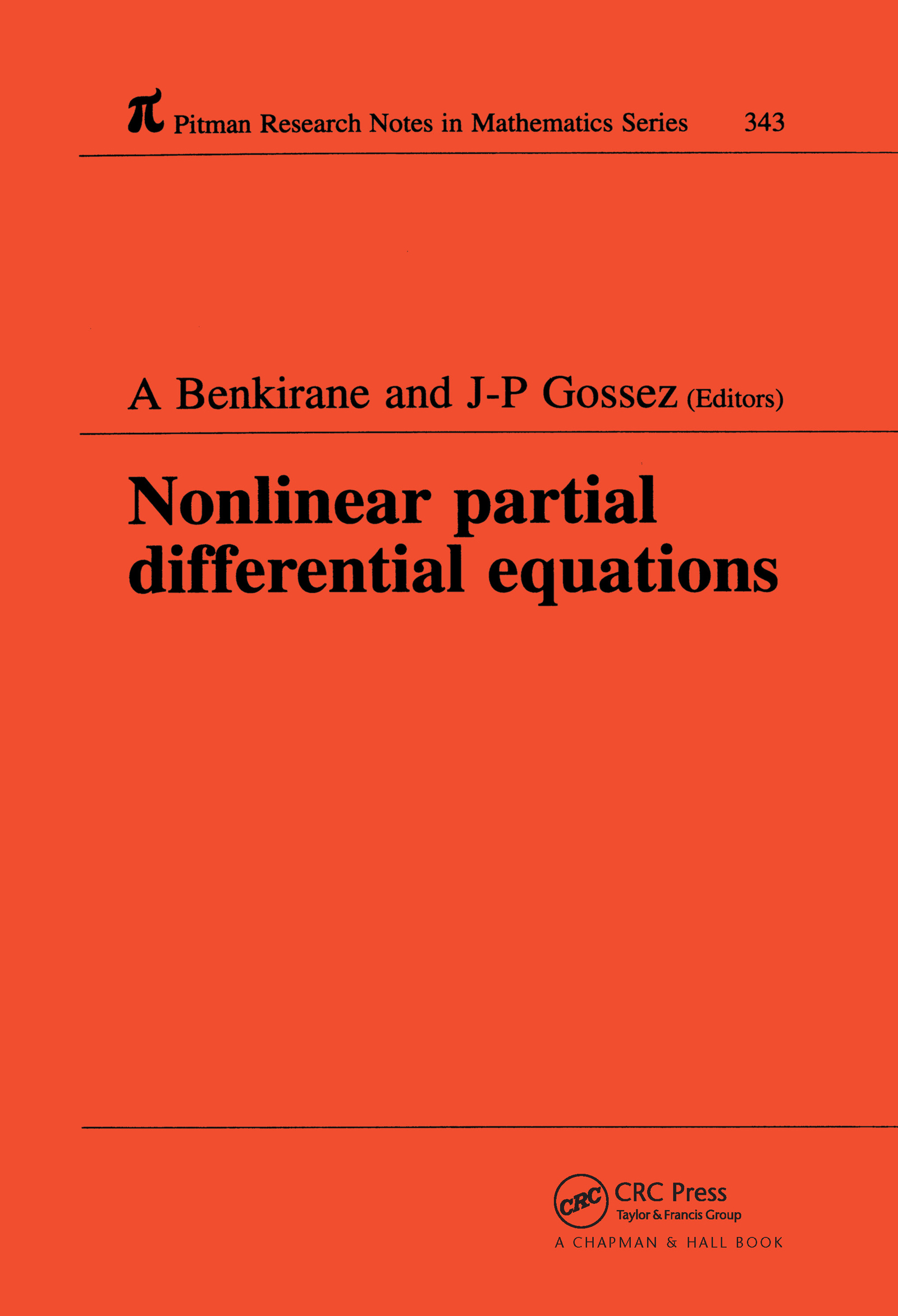Nonlinear Partial Differential Equations

1st Edition

Chapman and Hall/CRC

216 pages

Hardback: 9780582292130
pub: 1996-04-11
Currently out of stock
\$185.00
x

FREE Standard Shipping!

Description

This book presents a collection of selected contributions on recent results in nonlinear partial differential equations from participants to an international conference held in Fes, Morocco in 1994. The emphasis is on nonlinear elliptic boundary value problems, but there are also papers deveoted to related areas such as monotone operator theory, calculus of variations, Hamiltonian systems and periodic solutions. Some of the papers are exhaustive surveys, while others contain new results,published here for the first time. This book will be of particular interest to graduate or postgraduate students as well as to specialists in these areas.

On the second eigenvalue of the p-Laplacian

A theorem of H. Brezis and F.E. Browder type on Orlicz spaces and application

A result of maximum point localization for a semi-linear ellipticv pde

Existence results for semilinear equations with normal linear part and applications to

telegraph equations

The role of truncates in nonlinear Dirichlet problems in L1

Periodic solutions of second ordinary differential equations with a discontinuous nonlinearity

The range of sums of monotone operators and applications to Hammerstein inclusions and nonlinear complementarity problems

A priori estimates for positive solutions of semilinear elliptic systems via Hardy-Sobolev inequalities

On the minimisation of non quasiconvex integrals of the calculus of variations

Local solvability for complex quasi-linear equations

Strong nonlinear degenerated and singular elliptic problems

Some results about periodic solutions of second order Hamiltonian systems where the potential has indefinite sign

A note on nonresonance between consecutive eigenvalues for a semilinear elliptic problem

Cylindrical TE and TM modes in a self-focusing dielectric

Entropy solutions and the uniqueness problem for nonlinear second-order elliptic equations.